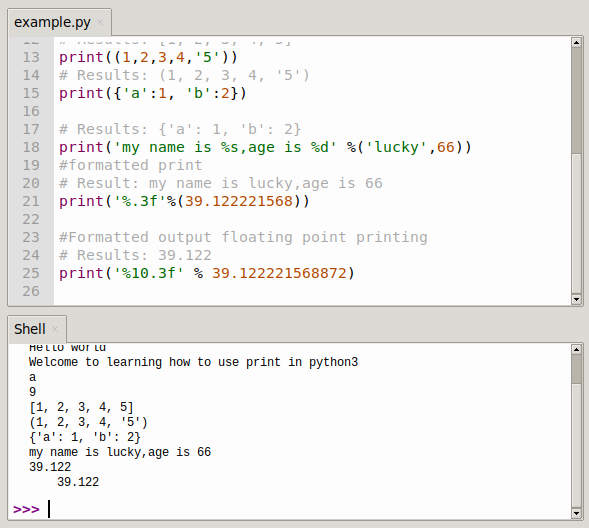# Python print() function

The print() function outputs text into the terminal (screen) or another output device. The message is usually a string, but you can print other things, whatever you want to show on the screen.

## Definition and usage

The `print()` function outputs whatever you pass as parameter.

``print("Hello world")``

The examples below demonstrate how you can use the print() function with Python 3. You can type them in the Python iteractive shell or run it as a .py file.

``````print('Welcome to learning how to use print in python3')

print('a')

# Outcome: a
print(9)

#Printing of numbers.
# Results: 9
print([1,2,3,4,5])

#Printing of lists
# Results: [1, 2, 3, 4, 5]
print((1,2,3,4,'5'))

# Results: (1, 2, 3, 4, '5')
print({'a':1, 'b':2})

# Results: {'a': 1, 'b': 2}
print('my name is %s,age is %d' %('lucky',66))

#formatted print
# Result: my name is lucky,age is 66
print('%.3f'%(39.122221568))

#Formatted output floating point printing
# Results: 39.122
print('%10.3f' % 39.122221568872) ``````more examples:

``````print('hello, world!')
print()
print("Hello, world!")
print()
print('Hello', 'World')
print()
print('hello', 'world', sep=', ', end='!')
print()
print()
print('goodbye, world', end='! \n')
print()
s = 'Hello'
length = len(s)
print("The length of %s is %d" % (s, length))
print()
pi = 3.141592653
print("Field width 10, precision 3:")
print('%10.3f' % pi)
print()
print("Read the field width or accuracy from the later tuple with *:")
print("pi = %. *f" % (3, pi))
print()
print("Fill in the blank with 0: ")
print('%010.3f' % pi)
print()
print("left-aligned: ")
print('%-10.3f' % pi)
print()
print("Show plus/minus sign: ")
print('%+f' % pi)
print()
print("print does not change lines:")
for i in range(10):
print(i, end='') # 0123456789
print()
print()
list = ['All','work','and','no','play']
print('-'.join(list))``````